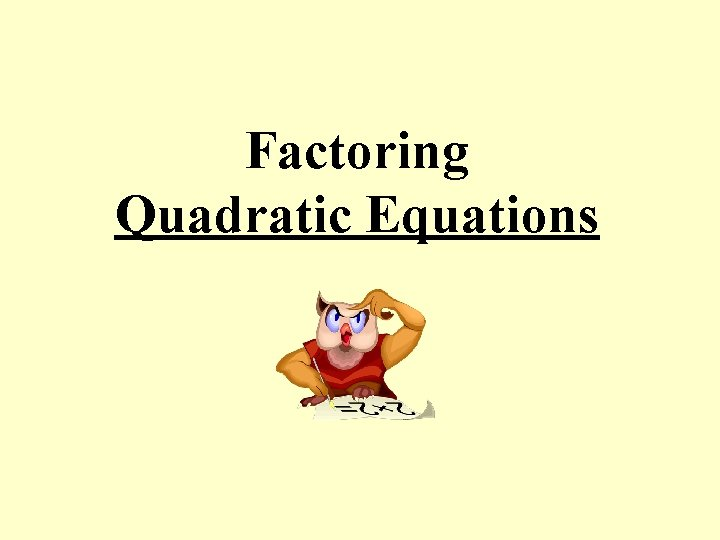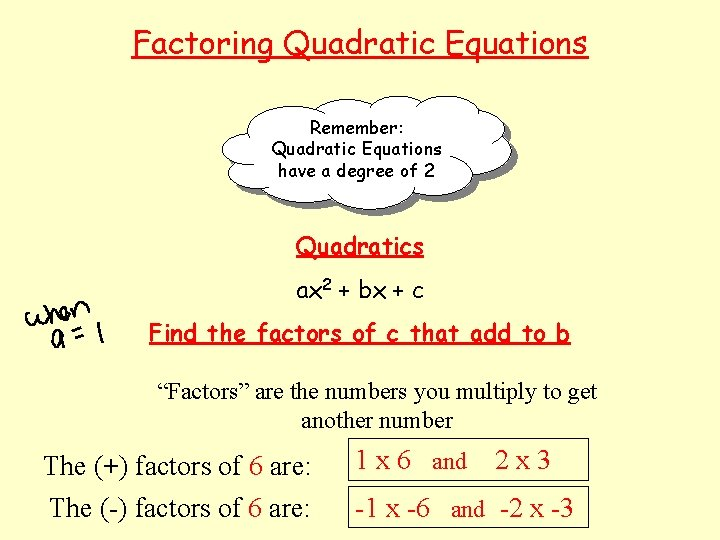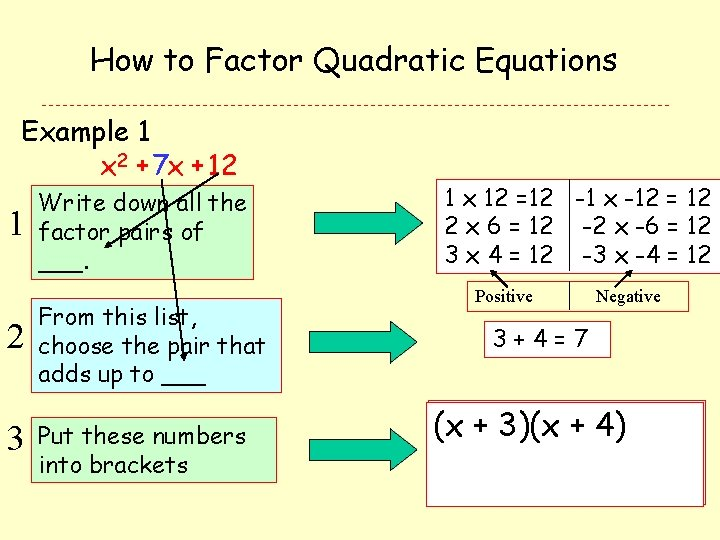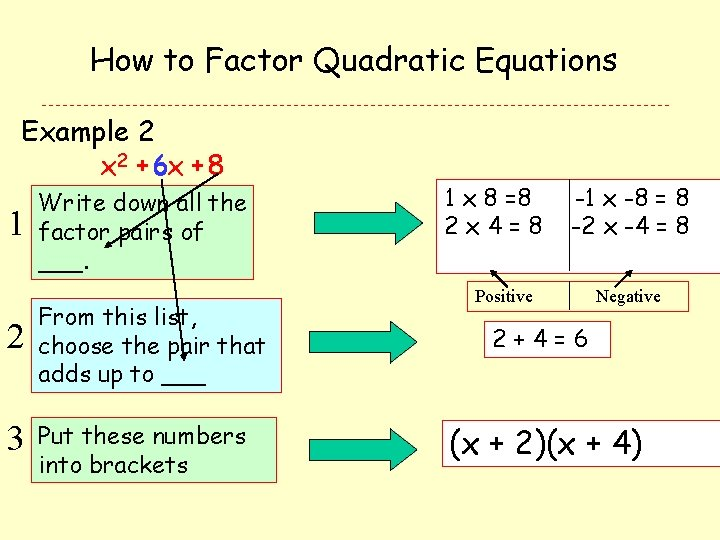• Slides: 8Factoring Quadratic Equations Remember: Quadratic Equations have a degree of 2 Quadratics ax 2 + bx + c Find the factors of c that add to b “Factors” are the numbers you multiply to get another number The (+) factors of 6 are: The (-) factors of 6 are: 1 x 6 and 2 x 3 -1 x -6 and -2 x -3How to Factor Quadratic Equations Example 1 x 2 + 7 x + 12 1 Write down all the factor pairs of ___. 2 From this list, choose the pair that adds up to ___ 3 Put these numbers into brackets 1 x 12 =12 -1 x -12 = 12 2 x 6 = 12 -2 x -6 = 12 3 x 4 = 12 -3 x -4 = 12 Positive Negative 3+4=7 )(x + 4)) (x + 3)(xHow to Factor Quadratic Equations Example 2 x 2 + 6 x + 8 1 Write down all the factor pairs of ___. 2 From this list, choose the pair that adds up to ___ 3 Put these numbers into brackets 1 x 8 =8 2 x 4=8 -1 x -8 = 8 -2 x -4 = 8 Positive Negative 2+4=6 (x + 2)(x + 4)How to Factor Quadratic Equations Example 2 x 2 -3 x -4 1 Write down all the factor pairs of ___. 2 From this list, choose the pair that adds up to ___ 3 Put these numbers into brackets -1 x 4 = -4 1 x -4 = -4 -2 x 2 = -4 2 x -2 = -4 1 + -4 = -3 (x - 4)(x + 1)1 x 2 + 5 x + 6 2 x 2 - x – 6 3 x 2 + 8 x + 12 4 x 2 + x – 12 5 x 2 - 8 x + 15 6 x 2 + 3 x – 21 7 x 2 - 3 x – 18 8 x 2 - 10 x – 24 9 x 2 + 8 x + 16 10 x 2 - 4 x – 601 x 2 + 5 x + 6 (x + 3)(x + 2) 2 x 2 - x – 6 (x – 3)(x + 2) 3 x 2 + 8 x + 12 (x + 2)(x + 6) 4 x 2 + x – 12 (x – 3)(x + 4) 5 x 2 - 8 x + 15 (x – 3)(x – 5) 6 x 2 + 3 x – 21 Does Not Factor 7 x 2 - 3 x – 18 (x – 6)(x + 3) 8 x 2 - 10 x – 24 (x - 12)(x + 2) 9 x 2 + 8 x + 16 (x + 4) 10 x 2 - 4 x – 60 (x - 10)(x + 6)Factor Solution: the following: x 2 - 5 xx+2 6 - =5 x(x+ -63) = (x - 2) (x - 3) (x - 2) Factor: Combine like terms: x(x - 2) -3(x - 2) x(x) + x(-2) - 3(x) - 3(-2) x 2 - 2 x - 3 x + 6 x 2 - 5 x + 6